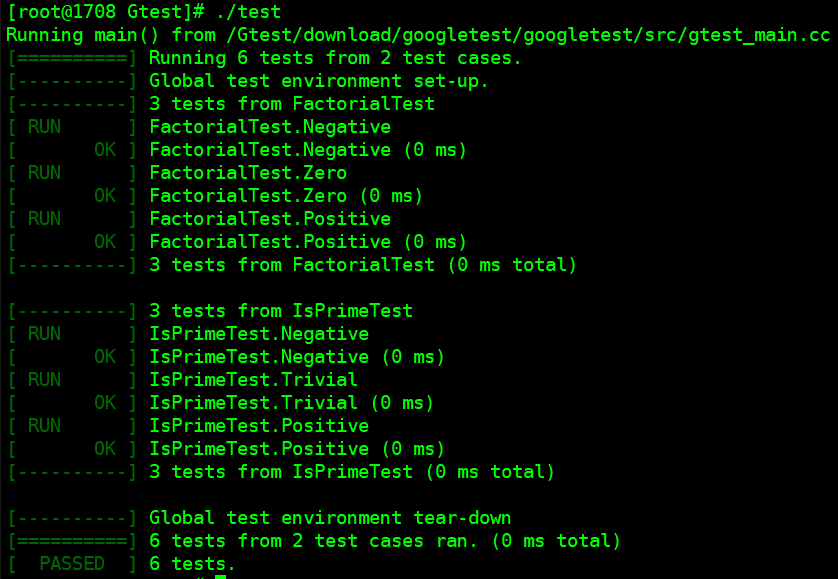# A quick introduction to Google test

sample.h#ifndef _SAMPLE_H_
#define _SAMPLE_H_

// Returns n! (the factorial of n).  For negative n, n! is defined to be 1.
int Factorial(int n);

// Returns true iff n is a prime number.
bool IsPrime(int n);

#endif
View Code

sample.c#include "sample.h"

// Returns n! (the factorial of n).  For negative n, n! is defined to be 1.
int Factorial(int n) {
int result = 1;
for (int i = 1; i <= n; i++) {
result *= i;
}

return result;
}

// Returns true iff n is a prime number.
bool IsPrime(int n) {
// Trivial case 1: small numbers
if (n <= 1) return false;

// Trivial case 2: even numbers
if (n % 2 == 0) return n == 2;

// Now, we have that n is odd and n >= 3.

// Try to divide n by every odd number i, starting from 3
for (int i = 3; ; i += 2) {
// We only have to try i up to the square root of n
if (i > n/i) break;

// Now, we have i <= n/i < n.
// If n is divisible by i, n is not prime.
if (n % i == 0) return false;
}

// n has no integer factor in the range (1, n), and thus is prime.
return true;
}
View Code

sample_unittest.c#include <limits.h>
#include "sample.h"
#include "gtest/gtest.h"
namespace {

TEST(FactorialTest, Negative) {
// This test is named "Negative", and belongs to the "FactorialTest"
// test case.
EXPECT_EQ(1, Factorial(-5));
EXPECT_EQ(1, Factorial(-1));
EXPECT_GT(Factorial(-10), 0);
}

TEST(FactorialTest, Zero) {
EXPECT_EQ(1, Factorial(0));
}

TEST(FactorialTest, Positive) {
EXPECT_EQ(1, Factorial(1));
EXPECT_EQ(2, Factorial(2));
EXPECT_EQ(6, Factorial(3));
EXPECT_EQ(40320, Factorial(8));
}

// Tests IsPrime()
TEST(IsPrimeTest, Negative) {
EXPECT_FALSE(IsPrime(-1));
EXPECT_FALSE(IsPrime(-2));
EXPECT_FALSE(IsPrime(INT_MIN));
}

TEST(IsPrimeTest, Trivial) {
EXPECT_FALSE(IsPrime(0));
EXPECT_FALSE(IsPrime(1));
EXPECT_TRUE(IsPrime(2));
EXPECT_TRUE(IsPrime(3));
}

TEST(IsPrimeTest, Positive) {
EXPECT_FALSE(IsPrime(4));
EXPECT_TRUE(IsPrime(5));
EXPECT_FALSE(IsPrime(6));
EXPECT_TRUE(IsPrime(23));
}
}  // namespace
View Code

g++ sample.c sample_unittest.c  -lgtest -std=c++11 -lgtest_main -lpthread -o testmain.c#include <gtest/gtest.h>
int main(int argc, char** argv){
return RUN_ALL_TESTS();
}
View Code

g++ sample.c sample_unittest.c  main.c -lgtest -std=c++11 -lpthread -o test

::testing::InitGoogleTest 方法的作用就是对框架进行初始化，必须在调用 RUN_ALL_TESTS 之前调用它。在代码中只能调用 RUN_ALL_TESTS 一次，因为多次调用会与框架的一些高级特性冲突，不支持这种做法。注意，RUN_ALL_TESTS自动地探测并运行用 TEST 宏定义的所有测试。在默认情况下，结果输出到标准输出。

Gtest选项

InitGoogleTest 函数接收传递给test infrastructure的参数，下面介绍常用参数#include "gtest/gtest.h"

TEST (DISABLE_SquareRootTest, PositiveNos) {
EXPECT_EQ (18.0, square-root (324.0));
EXPECT_EQ (25.4, square-root (645.16));
EXPECT_EQ (50.3321, square-root (2533.310224));
}

OR

TEST (SquareRootTest, DISABLE_PositiveNos) {
EXPECT_EQ (18.0, square-root (324.0));
EXPECT_EQ (25.4, square-root (645.16));
EXPECT_EQ (50.3321, square-root (2533.310224));
}
View Code1 FAILED TEST
YOU HAVE 1 DISABLED TEST
View Code

Assertions（断言）ASSERT_FLOAT_EQ (expected, actual)
ASSERT_DOUBLE_EQ (expected, actual)
ASSERT_NEAR (expected, actual, absolute_range)

EXPECT_FLOAT_EQ (expected, actual)
EXPECT_DOUBLE_EQ (expected, actual)
EXPECT_NEAR (expected, actual, absolute_range)
View CodeMath.cc(68): error: The difference between 2.00001 and 2.000011 is 1e-006, which exceeds
0.0000001, where
2.00001 evaluates to 2.00001,
2.000011 evaluates to 2.00001, and
0.0000001 evaluates to 1e-007.
View Code

Assertions会引发3种结果：success、Non-Fatal Failure、Fatal Failure

Non-Fatal Failure 和 Fatal Failure啥区别？

##### A test fixture classclass myTestFixture1: public ::testing::test {
public:
myTestFixture1( ) {
// initialization code here
}

void SetUp( ) {
// code here will execute just before the test ensues
}

void TearDown( ) {
// code here will be called just after the test completes
// ok to through exceptions from here if need be
}

~myTestFixture1( )  {
// cleanup any pending stuff, but no exceptions allowed
}

// put in any custom data members that you need
};
View CodeTEST_F (myTestFixture1, UnitTest1) {

.
}

TEST_F (myTestFixture1, UnitTest2) {

.
}
View Code

• 可以在构造函数或 SetUp 方法中执行初始化或分配资源。由用户选择具体方式。
• 可以在 TearDown 或析构函数例程中释放资源。但是，如果需要异常处理，那么只能在 TearDown 代码中进行，因为从析构函数中抛出异常会导致不确定的结果。
• 在以后的版本中，Google 断言宏可能会在平台上抛出异常。因此，为了便于维护，最好在 TearDown 代码中使用断言宏（assertion macros）。
• 不存在多个测试使用同一个test fixture。对于每个新的测试单元，框架创建一个新的test fixture。在上面代码中，由于要创建两个 myFixture1 对象，所以两次调用 SetUp 例程（请注意使用正确的拼写）。
posted @ 2018-12-22 15:57  克拉默与矩阵  阅读(...)  评论(...编辑  收藏